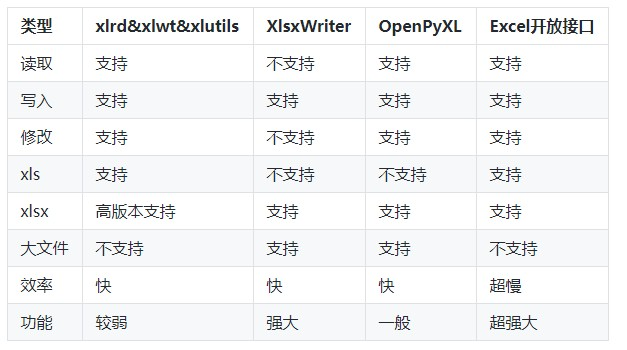Python 操作 Excel

## 常用工具## xlrd & xlwt & xlutils 介绍

xlrd&xlwt&xlutils 顾明思意是由以下三个库组成：

• xlrd：用于读取 Excel 文件；
• xlwt：用于写入 Excel 文件；
• xlutils：用于操作 Excel 文件的实用工具，比如复制、分割、筛选等；

# 安装

```pip install xlrd
pip install xlwt
pip install xlutils
```

# 导入

```import xlrd
import xlwt
import xlutils
```

# 读取excel

```# ----读取excel----
data= xlrd.open_workbook(excel_file)
```

# 读取sheet

```# ----读取sheet----
# 通过索引顺序获取sheet
table = data.sheets()

# 通过索引顺序获取sheet
table = data.sheet_by_index(0))

# 通过名称获取sheet
table = data.sheet_by_name("sheet")

# 返回book中所有sheet的名字
names = data.sheet_names()

# 传入索引或sheet名检查某个sheet是否导入完毕

# sheet名
table.name

# sheet列数
table.ncols

# sheet行数
table.nrows

```

# 读取sheet的行

```# 返回由rowx行中所有的单元格对象组成的列表
table.row(rowx)

# 获取rowx行第一个单元格的类型
# 0. empty（空的）,1 string（text）, 2 number, 3 date, 4 boolean, 5 error， 6 blank（空白表格）
table.row(rowx).ctype

# 获取rowx行第一个单元格的值
table.row(rowx).value

# 返回由rowx行中所有的单元格对象组成的列表
table.row_slice(self, rowx, start_colx=0, end_colx=None)

# 返回由rowx行中所有单元格的数据类型组成的列表
table.row_types(rowx, start_colx=0, end_colx=None)

# 返回由rowx行中所有单元格的数据组成的列表
table.row_values(rowx, start_colx=0, end_colx=None)

# 返回rowx行的有效单元格长度
table.row_len(rowx)

```

# 读取sheet的列

```#返回colx列中所有的单元格对象组成的列表
table.col(colx, start_rowx=0, end_rowx=None)

#返回colx列中所有的单元格对象组成的列表
table.col_slice(colx, start_rowx=0, end_rowx=None)

#返回colx列中所有单元格的数据类型组成的列表
table.col_types(colx, start_rowx=0, end_rowx=None)

#返回colx列中所有单元格的数据组成的列表
table.col_values(colx, start_rowx=0, end_rowx=None)

```

# 读取sheet的单元格

```# 返回单元格对象
cell = table.cell(rowx,colx)
# 单元格数据类型
# 0. empty（空的）,1 string（text）, 2 number, 3 date, 4 boolean, 5 error， 6 blank（空白表格）
cell.ctype
# 单元格值
cell.value
# 返回单元格中的数据类型
table.cell_type(rowx,colx)
# 返回单元格中的数据
table.cell_value(rowx,colx)
# 暂时还没有搞懂
table.cell_xf_index(rowx, colx)

```

# 写入excel

```# 使用xlutils将xlrd读取的对象转为xlwt可操作对象，table即上述xlrd读取的table
workbook = xlutils.copy(table)

# 或者如果你只是想创建一张空表
workbook = xlwt.Workbook(encoding = 'utf-8')

# 创建一个sheet
# 获取一个已存在的sheet
worksheet = workbook.get_sheet('sheet')

# 写入一个值，括号内分别为行数、列数、内容
worksheet.write(row, column, "memeda")

workbook.save('memeda.xls')

```

# 带样式写入示例

```workbook = xlwt.Workbook(encoding = 'utf-8')
style = xlwt.XFStyle()
font = xlwt.Font() # 创建字体
font.name = 'Arial'
font.bold = True # 黑体
font.underline = True # 下划线
font.italic = True # 斜体字
font.colour_index = 2 # 颜色为红色
style.font = font
worksheet.write(row, column, "memeda", style)
workbook.save('memeda.xls')

```

# 输出多种颜色字体

```import xlwt

workbook = xlwt.Workbook(encoding='utf-8')

def get_style(i):
style = xlwt.XFStyle()
font = xlwt.Font()  # 创建字体
font.colour_index = i
style.font = font
return style

for i in range(0, 100):
sheet.write(i, 0, "memeda", get_style(i))
workbook.save('memeda.xls')
```• 发表于 2020-07-13 17:38
• 阅读 ( 157 )
• 分类：python

## 作家榜 »

1.omicsgene 327 文章
2.安生水 217 文章
3.Daitoue 167 文章
4.生物女学霸 120 文章
5.CORNERSTONE 72 文章
6.生信老顽童 48 文章
7.红橙子 47 文章
8.landy 37 文章